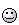# Energy transition of LiCl from flame test

• Jpyhsics
Im not sure the bohr equation would work since it is for atoms with a single electron.In summary, the Homework Statement is that the LiCl flame test is red. The process would be emission, as photons are emitted when energy is released. The lowest energy transition would be from n=2 to n=1, which would give us the greatest wavelength of visible light. Lyman, Balmer, Paschen, Brackett, and Pfund are some of the series that would be applicable to this information.f

## Homework Statement

LiCl flame test is red.
a) define what process
b)and transition between which energy levels?

2. The attempt at a solution
a)I believe that the process would be emission, as photons are emitted when energy is released
b)n=2 to n=1
I assumed that that would be the lowest energy transition, which would give us the greatest wavelength of visible light. My only question is why in the hydrogen spectrum is the transition from n=3 to n=2 red light, or anything to transition to n=2 is visible, whereas here would it be different as lithium has 3 e-? Would lithium also emit visible light from transition to n=2? So would n=3 to n=2 be the correct answer?

b)n=2 to n=1
I assumed that that would be the lowest energy transition,
Is your "assumption" based on fact; small quantum numbers imply larger or smaller energy changes?

Is your "assumption" based on fact; small quantum numbers imply larger or smaller energy changes?
Well in our lecture our prof said that a small energy change is equal to small frequency and longer wavelength, and I know red is a longer wavelength in the visible spectrum.

Well in our lecture our prof said that a small energy change is equal to small frequency and longer wavelength, and I know red is a longer wavelength in the visible spectrum.
Would n=2 to n=1 be correct?

Lyman...Balmer...Paschen...Brackett ...Pfund...? Ring any bells?

So far nobody asked: what is Z for lithium?

Lyman...Balmer...Paschen...Brackett ...Pfund...? Ring any bells?
so would it be n=3 to n=2? since the balmer series is visible light and all transitions to n=2?

So far nobody asked: what is Z for lithium?
The Z is 3

So can you apply hydrogen atom series directly to the energies of the lithium atom transitions?

So can you apply hydrogen atom series directly to the energies of the lithium atom transitions?
No...?

No...?
So what would I have to use?

the balmer series
is for hydrogen. Think transition energy.

is for hydrogen. Think transition energy.
Well energy wise I am thinking that n=2 to n=1 transition would emit the least amount of energy, which would indicate a longer wavelength. So would that be right?

n=2 to n=1 transition would emit the least amount of energy,
"Least?""Red" is a reasonably well defined range of wavelengths, calculate if the 2→1 transition falls in that range.

"Red" is a reasonably well defined range of wavelengths, calculate if the 2→1 transition falls in that range.
Or would this have to do with the sublevels? so the electron goes from 2p to 2s?

I am a little confused at what everyone is trying to get at.

"Least?"Then what would be considered the lowest energy transition?

the lowest energy transition?
The "last" Δn=1 transition before the "continuum," ∞-1 to ∞-2, change in energy levels is infinitesimal.I told you - rather precisely - what to check. Have you tried?

I told you - rather precisely - what to check. Have you tried?
Im not sure the bohr equation would work since it is for atoms with a single electron.